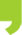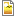## You are here

• You are here:
• Home > Events > Basics of Biostatistics 2021: Statistical Analysis applied to Research Data

# Basics of Biostatistics 2021: Statistical Analysis applied to Research Data

##Basics of Biostatistics 2021: Statistical Analysis applied to Research DataDownload poster HERE

23/04/2021
Add to Calendar

## Basics of Biostatistics 2021: Statistical Analysis applied to Research Data

Description:

The course will give an overview of important concepts and methods used to analyse “Biomedical data”. The emphasis will be on the understanding of statistical concepts and their interpretation in a research framework. After a general introduction on probability theory and statistical inference, an emphasis will be made on common statistical methods. Particular cases will be used as illustrative examples.

The course will be composed of face-to-face theoretical lectures combined with practical sessions and additional short homework assignment.

Duration: 10 sessions (total 20h)
Schedule:  Each Friday from 23rd April (9:00-12:00)  to 9th July 2021
Time: 9:30-11:30am
Where: Zoom
Requirements: Participants need to have basic R programing skills
Application deadline: 16th of March 2021
Registration HERE

Title Type Date from 09:30-11:30
Introduction. Descriptive statistics. Probability and distributions. Sampling distribution. Confidence intervals Theory 23rd April (9:00-12:00)
Hypothesis testing. The t-test. Theory 30th April
Exercises and Hands on sessions 1-2. Practicum 7th May
Analysis of variance. One-way ANOVA Theory

14th May

ANOVA. Multiple comparison procedures. Two-way ANOVA. Theory 21st May
Hands on sessions 3-4. Practicum 28th May
Correlation and regression Theory 4th June
Multiple regression analysis. Partial correlation. Theory 11th June
Hands on sessions 7-8 Practicum 18th June
Effect Size and a summary of inference methods. Theory 2nd July

At the end of the course, the students will be able to:

• Express a scientific question in a mathematical formulation possible to analyse with statistical method.
• To be  confident about sampling methods and their impact on the quality of the data.
• Think on the basis of experimental design: replication, randomization and stratification.
• Think about the appropriate statistical method given the data we have (dependent and/or independent variable, continuous or categorical variables).
• Have a deep understanding of the meaning and interpretation of a test statistic and how to construct own statistic given data.
• Have a correct understanding of key words in basic statistic: null hypothesis, alternative hypothesis, error type I, error type II, statistical power, observational study, experimental study, causation and correlation, sampling error, random error, systematic error, sampling distribution, p-value, statistical and practical significance, effect size, statistical power.
• Correct interpretation of the result of a statistical test and been aware of the limitations of the applied methods.internal_training_bacis_of_biostatistics_2021.png